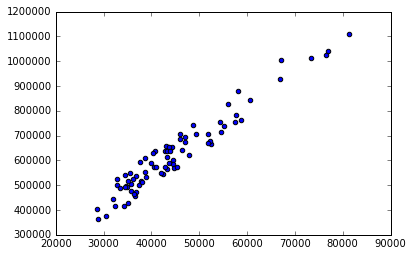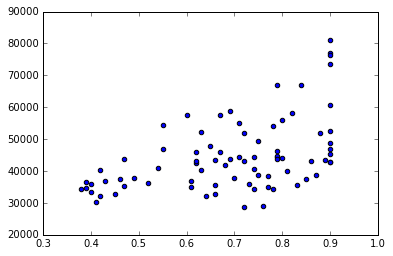• No products in the cart.

# 204.1.3 Practice : Regression Line Fitting

Link to the previous post : https://statinfer.com/204-1-2-regression-in-python/

In the last post we went through concept of Regression. In this post we will try to implement and practice Linear regression.

### Practice : Regression Line Fitting

• Dataset: AirPassengers\AirPassengers.csv
• Find the correlation between Promotion_Budget and Passengers
• Draw a scatter plot between Promotion_Budget and Passengers. Is there any any pattern between Promotion_Budget and Passengers?
• Build a linear regression model on Promotion_Budget and Passengers.
• Build a regression line to predict the passengers using Inter_metro_flight_ratio
In :
```import pandas as pd
air.shape
```
Out:
`(80, 9)`
In :
```air.columns.values
```
Out:
```array(['Week_num', 'Passengers', 'Promotion_Budget',
'Service_Quality_Score', 'Holiday_week',
'Delayed_Cancelled_flight_ind', 'Inter_metro_flight_ratio',
In :
```air.head(5)
```
Out:
Week_num Passengers Promotion_Budget Service_Quality_Score Holiday_week Delayed_Cancelled_flight_ind Inter_metro_flight_ratio Bad_Weather_Ind Technical_issues_ind
0 1 37824 517356 4.00000 NO NO 0.70 YES YES
1 2 43936 646086 2.67466 NO YES 0.80 YES YES
2 3 42896 638330 3.29473 NO NO 0.90 NO NO
3 4 35792 506492 3.85684 NO NO 0.40 NO NO
4 5 38624 609658 3.90757 NO NO 0.87 NO YES
In :
```# Find the correlation between Promotion_Budget and Passengers
import numpy as np
np.corrcoef(air.Passengers,air.Promotion_Budget)
```
Out:
```array([[ 1.        ,  0.96585103],
[ 0.96585103,  1.        ]])```
In :
```# Draw a scatter plot between   Promotion_Budget and Passengers. Is there any any pattern between Promotion_Budget and Passengers?

import matplotlib.pyplot as plt
%matplotlib inline

plt.scatter(air.Passengers, air.Promotion_Budget)
```
Out:
`<matplotlib.collections.PathCollection at 0x90bda20>`In :
```#Build a linear regression model and estimate the expected passengers for a Promotion_Budget is 650,000
##Regression Model  promotion and passengers count

from sklearn.linear_model import LinearRegression
lr = LinearRegression()
lr.fit(air[["Promotion_Budget"]], air[["Passengers"]])
predictions = lr.predict(air[["Promotion_Budget"]])
```
In :
```import statsmodels.formula.api as sm
model = sm.ols(formula='Passengers ~ Promotion_Budget', data=air)
fitted1 = model.fit()
```
In :
```fitted1.summary()
```
Out:
Dep. Variable: R-squared: Passengers 0.933 OLS 0.932 Least Squares 1084. Wed, 27 Jul 2016 1.66e-47 11:48:26 -751.34 80 1507. 78 1511. 1 nonrobust
coef std err t P>|t| [95.0% Conf. Int.] 1259.6058 1361.071 0.925 0.358 -1450.078 3969.290 0.0695 0.002 32.923 0.000 0.065 0.074
 Omnibus: Durbin-Watson: 26.624 1.831 0 5.188 -0.128 0.0747 1.779 2.67e+06
In :
```# Build a regression line to predict the passengers using Inter_metro_flight_ratio

plt.scatter(air.Inter_metro_flight_ratio,air.Passengers)
```
Out:
`<matplotlib.collections.PathCollection at 0xb13f2b0>`In :
```import sklearn as sk

from sklearn.linear_model import LinearRegression
lr = LinearRegression()
lr.fit(air[["Inter_metro_flight_ratio"]], air[["Passengers"]])
```
Out:
`LinearRegression(copy_X=True, fit_intercept=True, n_jobs=1, normalize=False)`
In :
```predictions = lr.predict(air[["Inter_metro_flight_ratio"]])
```
In :
```import statsmodels.formula.api as sm
model = sm.ols(formula='Passengers ~ Inter_metro_flight_ratio', data=air)
fitted2 = model.fit()
```
In :
`fitted2.summary()`
Out:
Dep. Variable: R-squared: Passengers 0.242 OLS 0.232 Least Squares 24.90 Wed, 27 Jul 2016 3.58e-06 11:48:27 -848.30 80 1701. 78 1705. 1 nonrobust
coef std err t P>|t| [95.0% Conf. Int.]
Intercept 2.044e+04 4993.747 4.093 0.000 1.05e+04 3.04e+04
Inter_metro_flight_ratio 3.507e+04 7027.768 4.990 0.000 2.11e+04 4.91e+04
 Omnibus: Durbin-Watson: 10.172 1.385 0.006 10.098 0.822 0.00641 3.573 9.48

The next post is on how good is my regression line.

Link to the next post : https://statinfer.com/204-1-4-how-good-is-my-regression-line/

21st June 2017